$$\def\loading{......LOADING......Please Wait......} \def\RR{\bf R} \def\real{\mathbb{R}} \def\bold#1{\bf #1} \def\d{\mbox{Cord}} \def\hd{\widehat \mbox{Cord}} \DeclareMathOperator{\cov}{cov} \DeclareMathOperator{\var}{var} \DeclareMathOperator{\cor}{cor} \newcommand{\ac}{\left\{#1\right\}} \DeclareMathOperator{\Ex}{\mathbb{E}} \DeclareMathOperator{\diag}{diag} \newcommand{\bm}{\boldsymbol{#1}} \def\wait{......LOADING......Please Wait......}$$

## Causal Dynamic Networks: ODE Network Modeling of fMRI

### Xi (Rossi) LUO

Brown University
Department of Biostatistics
Center for Statistical Sciences
Computation in Brain and Mind
Brown Institute for Brain Science
Brown Data Science Initiative
ABCD Research Group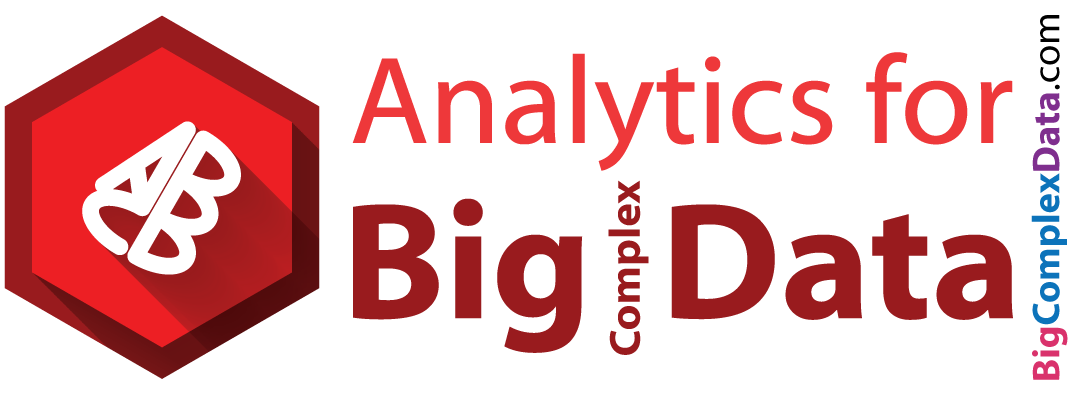CMStatistics, Pisa Italy
December 16, 2018

Funding: NIH R01EB022911, P20GM103645, P01AA019072, P30AI042853; NSF/DMS (BD2K) 1557467

## Co-AuthorsXuefei Cao
Brown Applied MathBjörn Sandstede
Brown Applied Math

# Slides viewable on web: bit.ly/cmstat18

## fMRI Experiments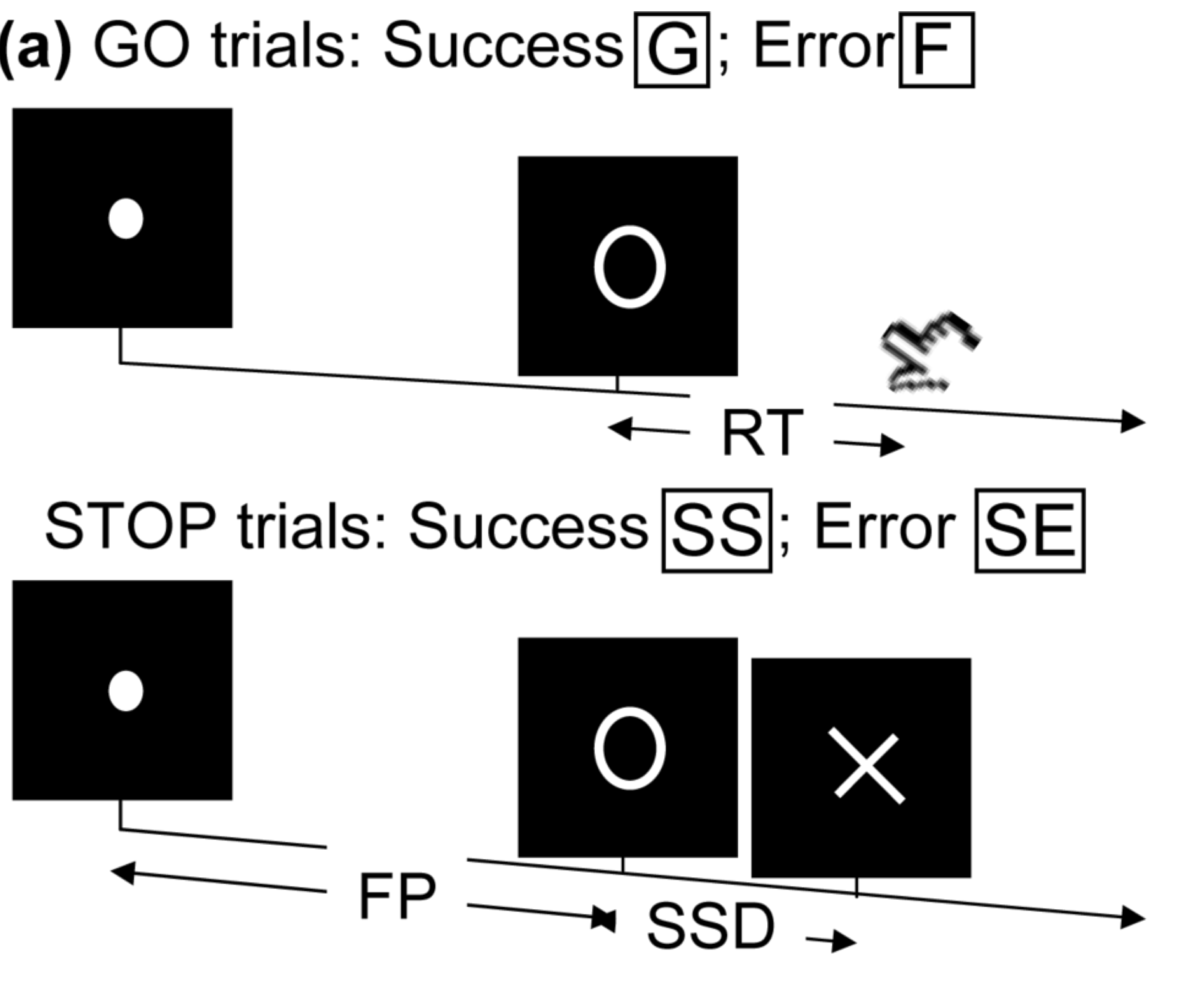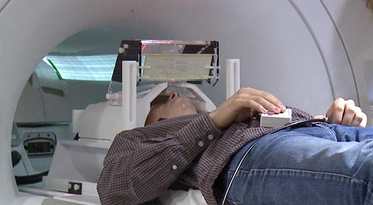• press button if "go";
• withhold pressing if "stop"
• Resting-state: "do nothing" during scanning

Goal: infer task-related brain activation and connectivity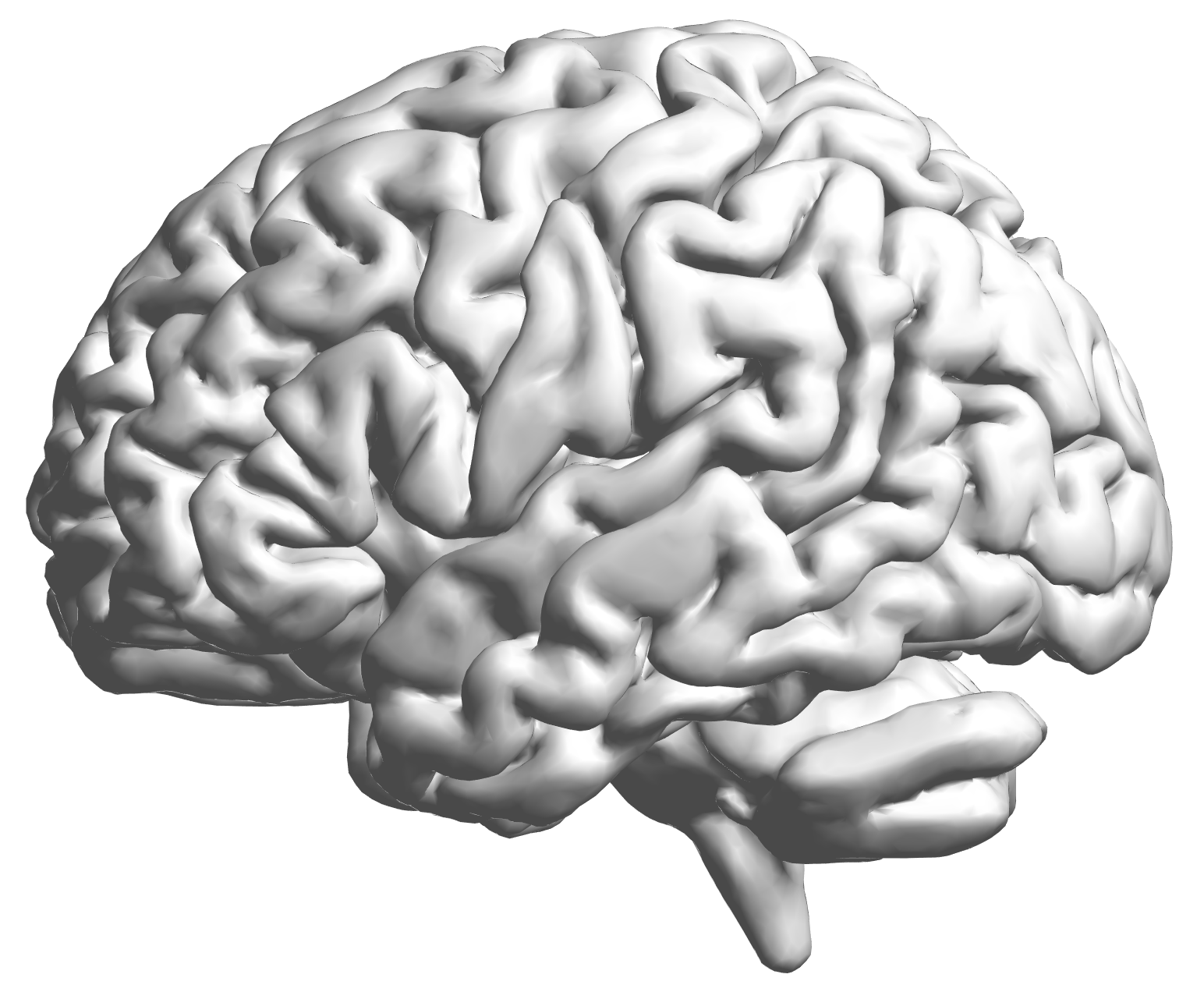fMRI data: blood-oxygen-level dependent (BOLD) signals from each cube/voxel (~millimeters), $10^5$ ~ $10^6$ voxels in total.

## Conceptual Model with Stimulus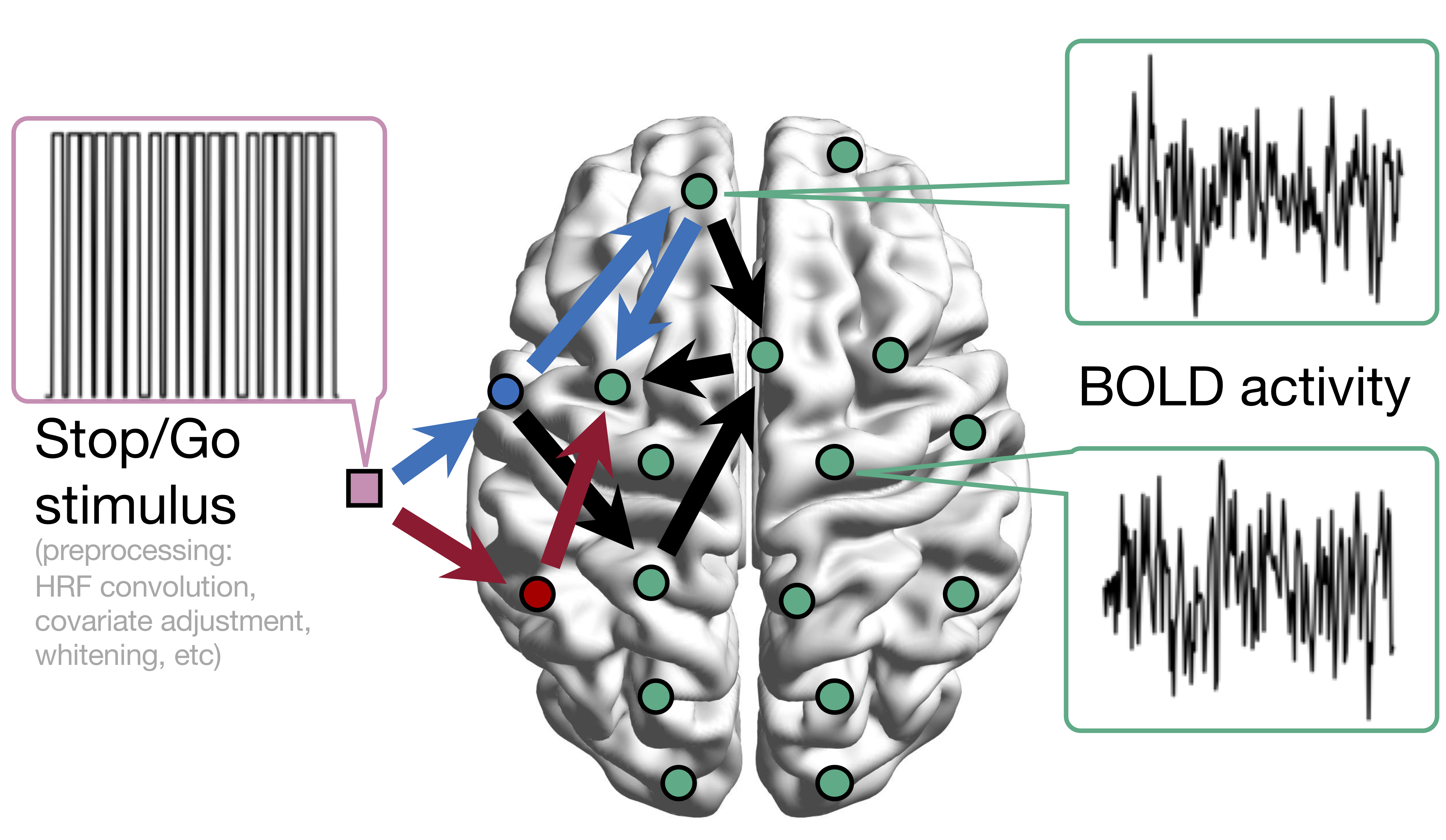Sci Goal: infer intrinsic connections, "Go"-task related connections, "Stop" connections

## Some Existing Methods and Limitions

• Functional (nondirectional) connectivity:
• Correlations
• PCA, independent component analysis (ICA) Calhoun, Guo, and colleagues
• Graphical models (inverse covariance)
• Bayesian methods Bownman, Guindani, Vannucci, Zhang, and colleagues
• Effective (directional) connectivity:
• Granger causality, autoregressive modelsDing, Hu, Ombao, and colleagues
• Structural equation models
• Limitations:biophysical interpretability $\propto$ scalability${}^{-1}$
• Fail to model task-depend connections/activations
• Connections unlikely to be causal/neuronal
• Some are hard to scale to large networks

## Dynamic Causal Modeling (DCM)

• Proposed by Friston et al, 2003 (> 3000 citations)
• System approach to address previous limitations:
• Latent neuronal states: a network ODE model
• From neuronal states to observed BOLD signals: another ODE
• (Bayesian) priors for model parameters
• Bayes factors for comparing a few candidate models
• DCM essentially unchanged for the past 15 yrs Friston et al, 17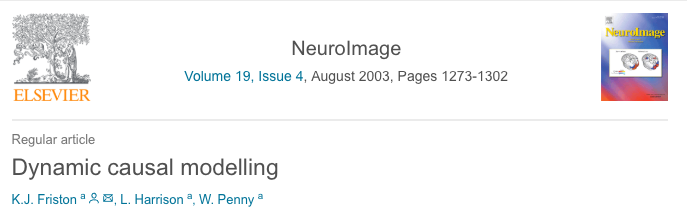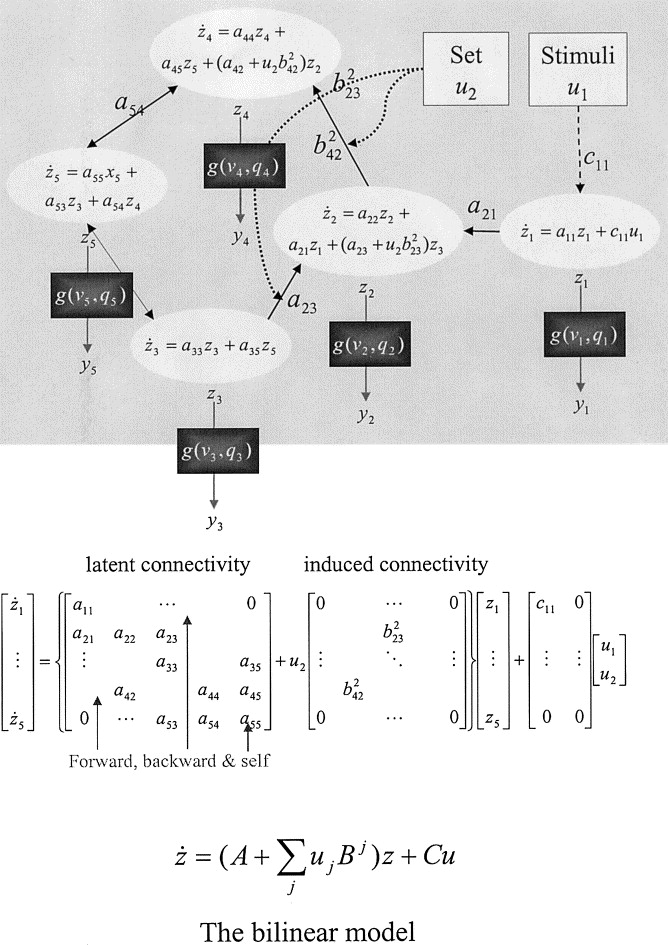• Neuronal/causal connections
• Model brain activations (and non-stationary time series)
• Limitations:
• Computationally expensive
• Bayesian model comparison over exponentially many models
• Model performance depends on priors Frassle et al, 15
• Hard to scale (~10 nodes), some successes for simplified models
• Mostly for hypothesis validation, not data driven

# Our CDN Model and Method

## Causal Dynamic Networks

• A two-level model
• 1. DCM neuronal state model (latent $\bm{x}$, stimulus $\bm{u}$): $$\frac{d\bm{x}(t)}{dt}=\bm{A}\bm{x}(t)+\sum_{j}u_j(t)\bm{B_j}\bm{x}(t)+\bm{C}\bm{u}(t)$$
• 2. BOLD data model (data $\bm{y}$, noise $\bm{\epsilon}$) at discrete $t_i$: $$\bm{y}(t_i) =\int h(s)\bm{x}(t_i-s) ds + \bm{\epsilon} (t_i)$$
• $h$ hemodynamic response function
• $\bm{A}$ intrinsic connection matrix, $\bm{B}$ task-dependent connection tensor, $\bm{C}$ stimulus activation matrix

## Functional/Dynamic Data Analysis

• Usually, observed data model $$y(t) = x(t) + \epsilon(t)$$ and latent $x(t)$ follows an ODE model of interest
• Various approaches for estimating the ODE parameters: nonlinear least squares Xue, Miao, Wu, 10, two-stage smoothing Varah, 82, principal differential analysis Ramsey, 96, Bayesian Girolami, 08, EcoG Zhang et al, 15
• The observed data model not applicable to fMRI
• For example, two-stage smoothing approaches not directly applicable to BOLD convolutions: $$y(t) = \int x(t-u)h(u) du + \epsilon(t)$$

## Hemodynamic Response Function (HRF)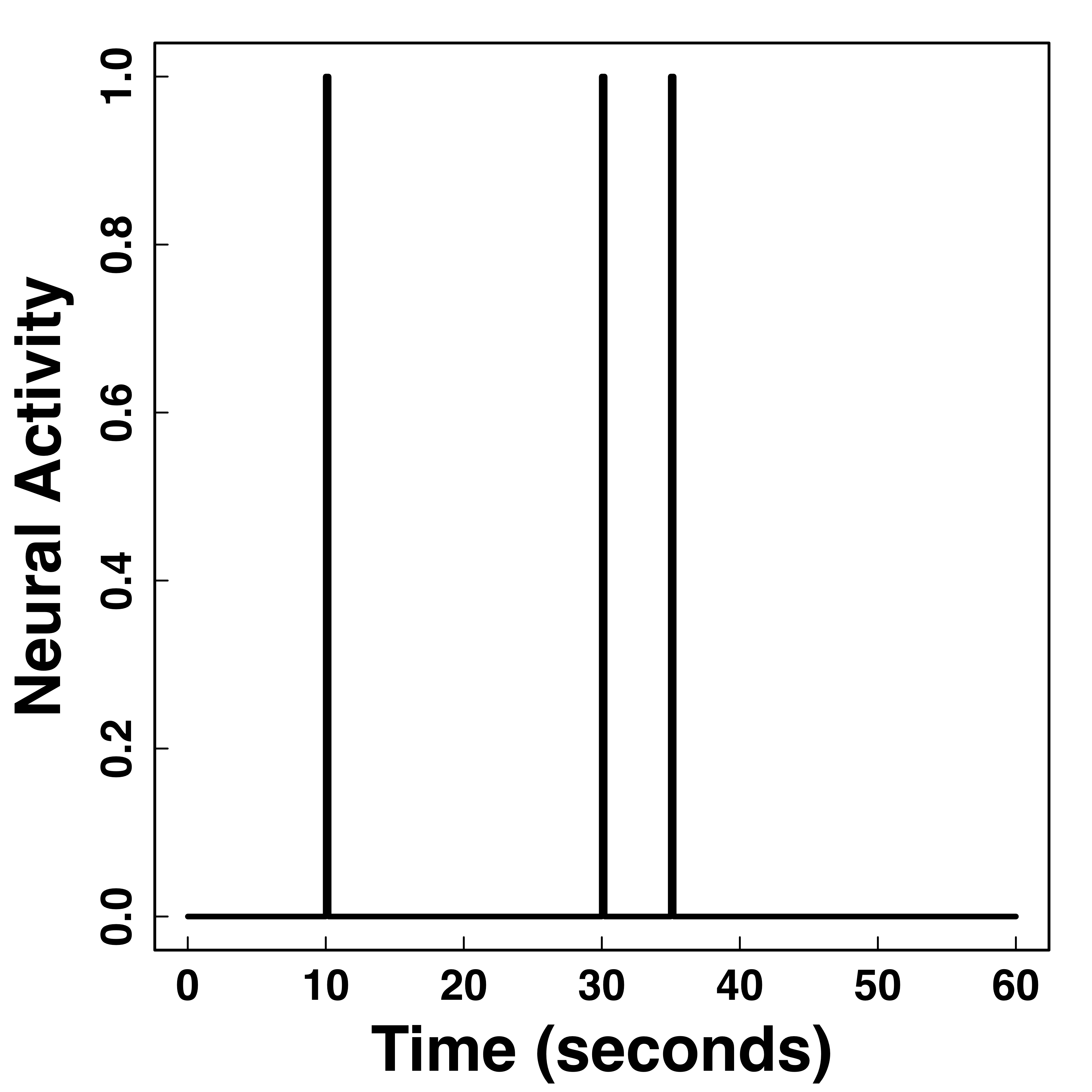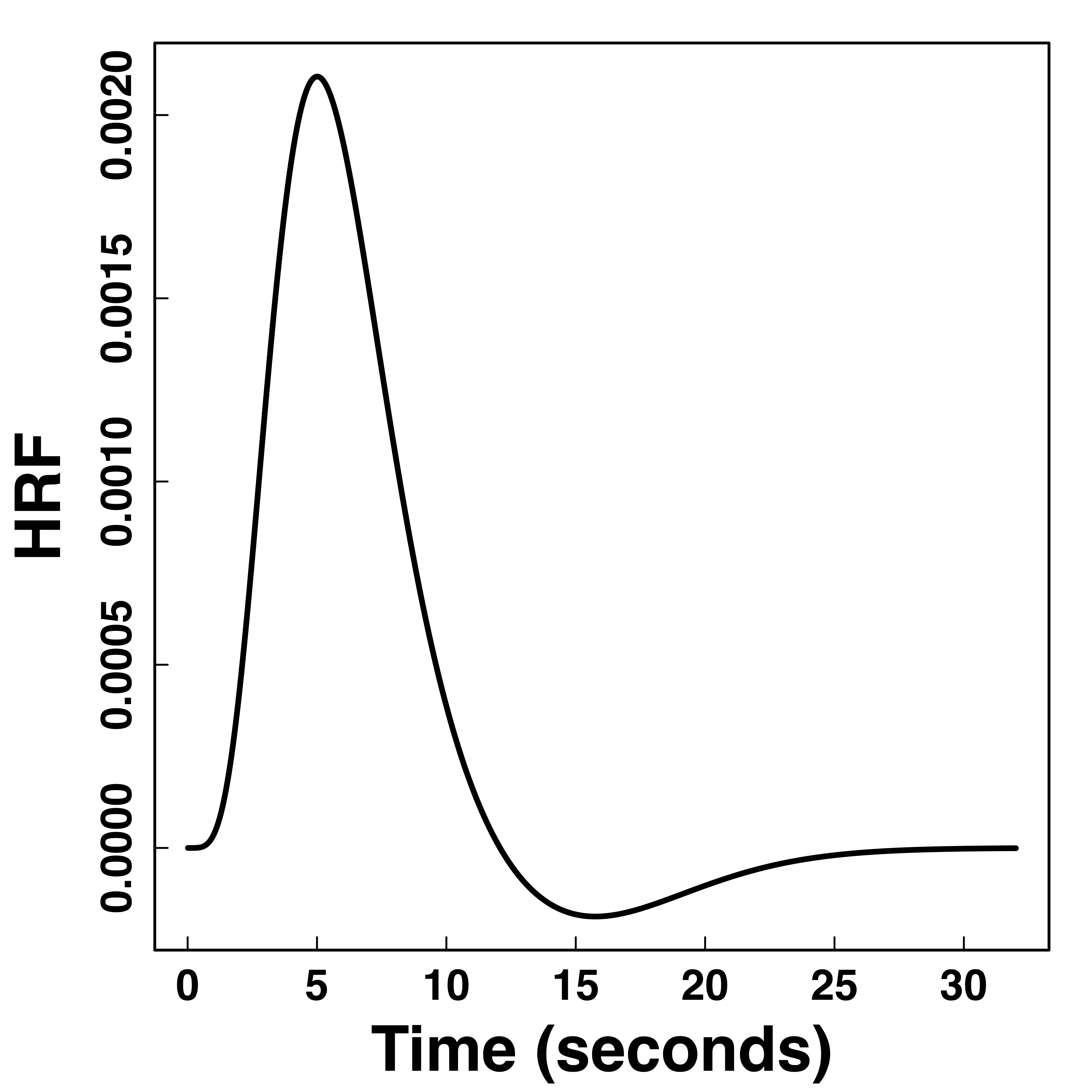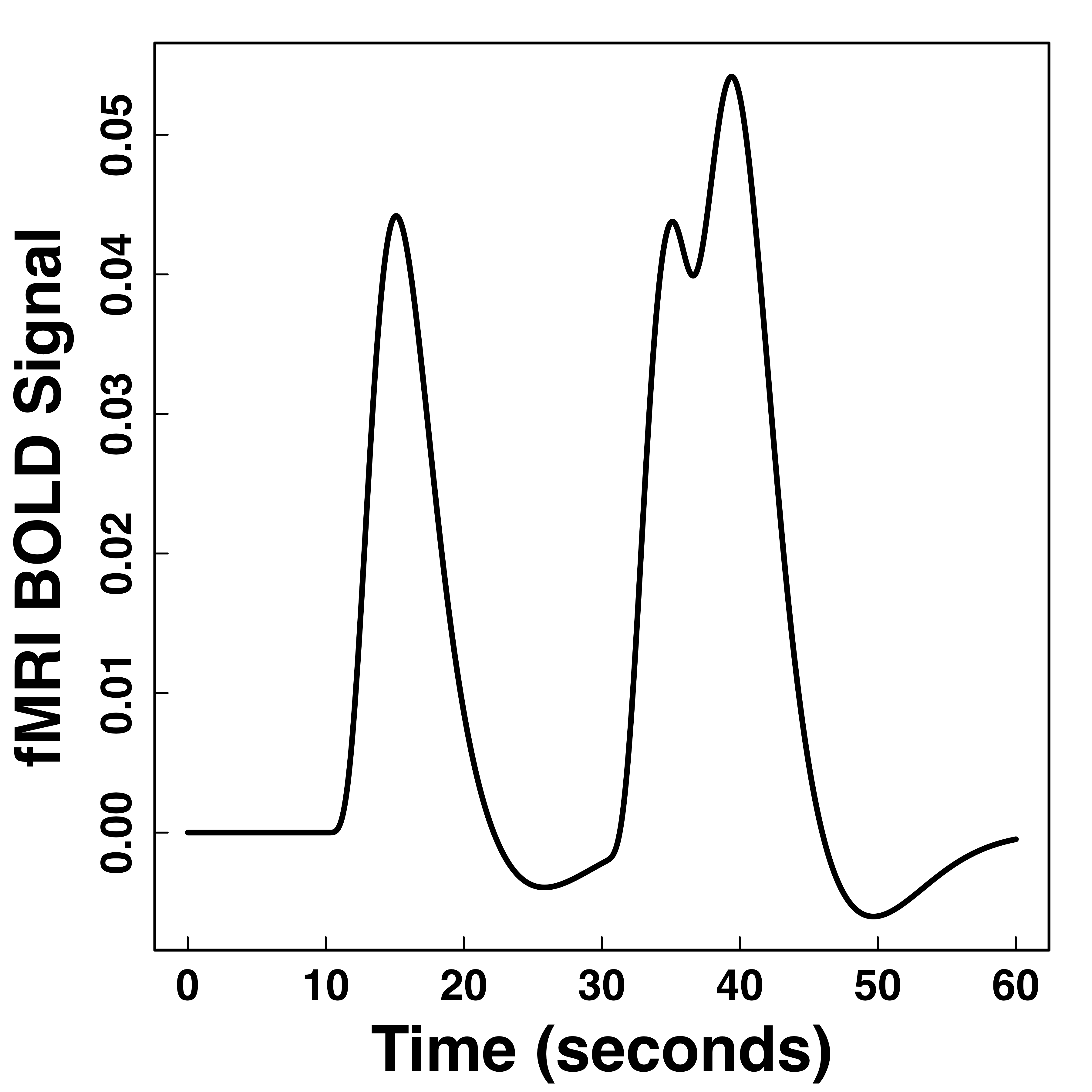fMRI responses last long (~30 seconds) after neural activities

"Smooth" BOLD far from neuronal activity

## Method

• An optimization-based approach
• Minimize the following $\begin{multline*} l(\bm{x},\bm{\theta})=\sum_{t_i} \| \bm{y}(t_i)-h \star \bm{x}(t_i)) \|^2 \\ +\lambda\int \left \| \frac{d \bm{x} (t) } {dt} - (A \bm{x}(t)+\sum_{j} u_j(t) \bm{B_j} \bm{x}(t)+ \bm{C}\bm{u}(t)) \right\|^2 dt \end{multline*}$
• Balancing data fitting errors and ODE fitting errors
• Plug in basis-expansion of $\bm{x}(t) = \bm{\Gamma} \bm{\Phi}(t)$
• Allows convolution (vs two-stage smooth approach)
• Computationally fast to allow Bootstrap inference

## Algorithm

• Prove conditional convexity of $O(J d^2)$ parameters
• Iterative block coordinate descent algorithm
• Prove explicit update formulas (no numerical optimization algorithms needed)

## Special Case: Resting-state fMRI

• Set our parameter $\bm{B}$ and $\bm{C}$ to zero
• Only fit intrinsic connection $\bm{A}$
• Can fit much larger networks

# Simulations

## Simulation: vs GCA/VAR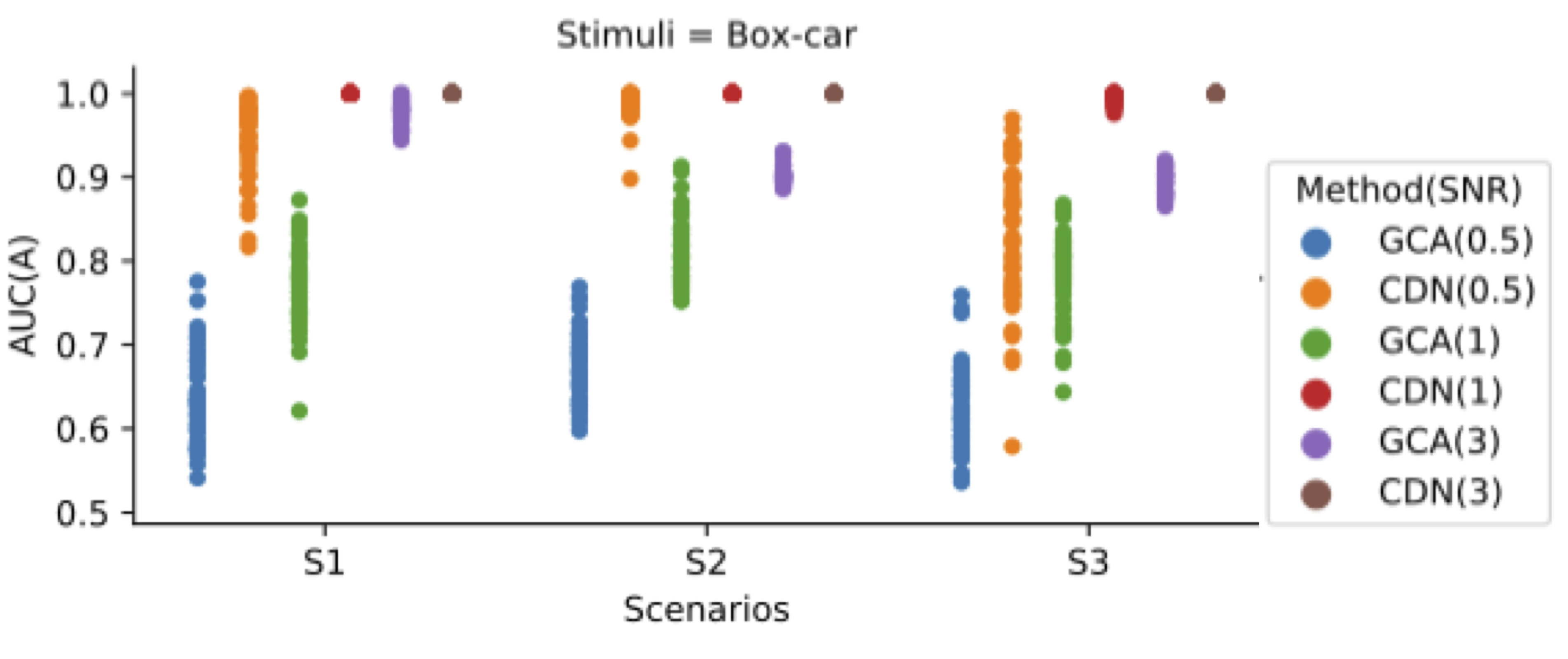Our CDN yields higher network recovery accuracy than Granger Causality Analysis (GCA, aka vector autoregressive models)

## Simulation: vs DCM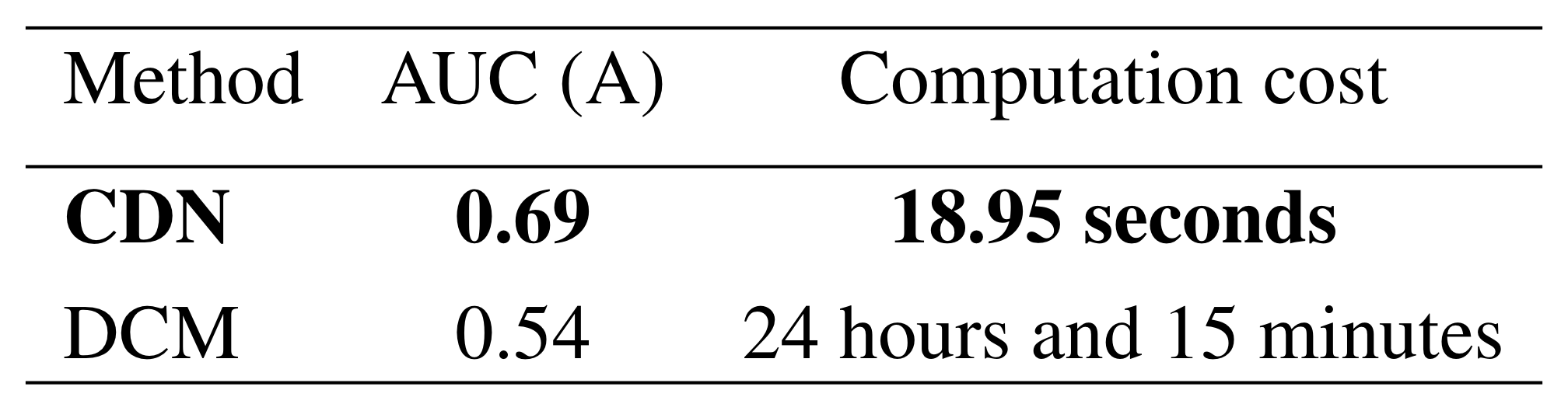Our CDN yields higher accuracy using only a fraction of the computation time of DCM

## Uncovering Neuronal States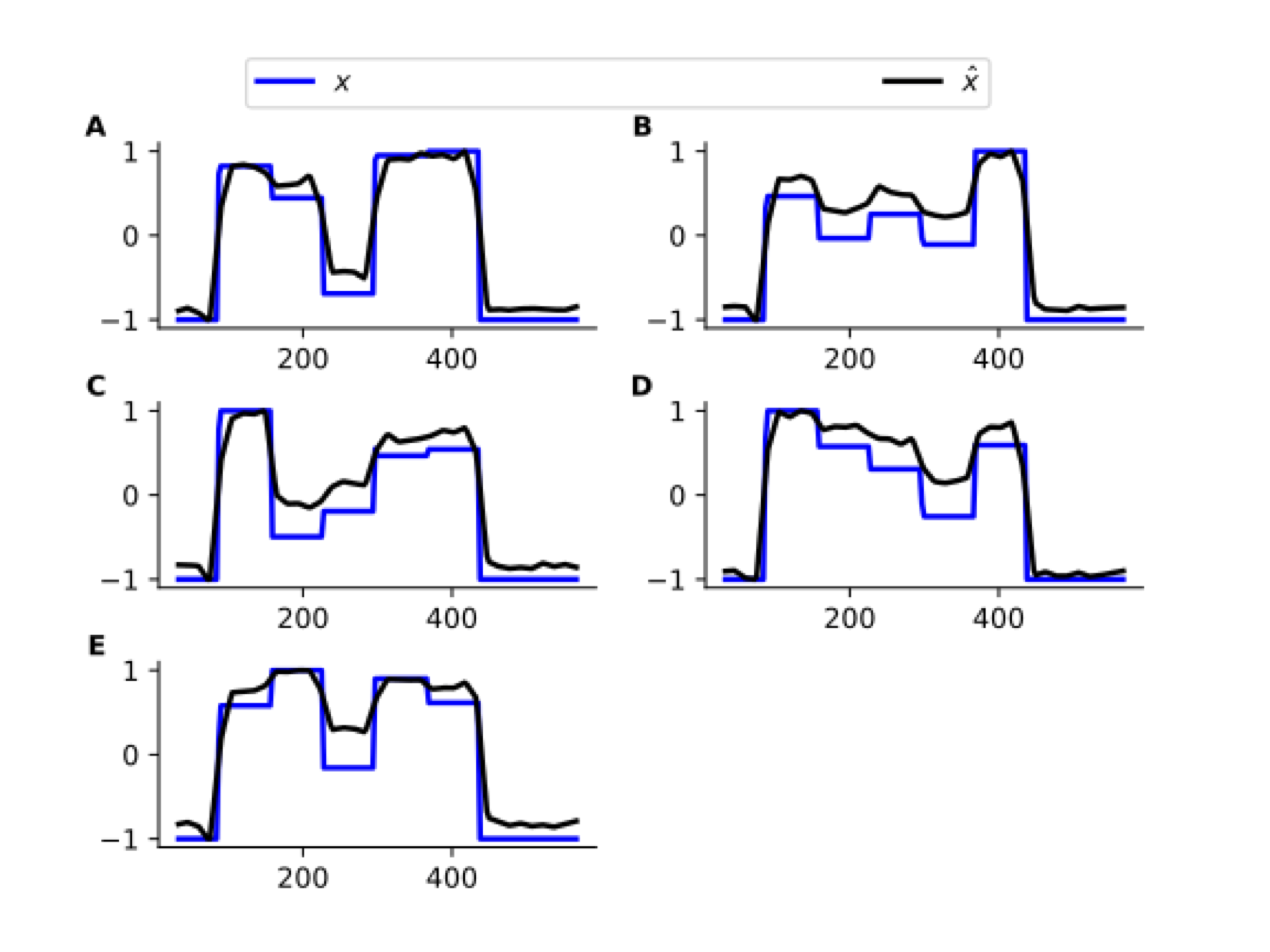Decent recovery of (latent) neuronal states

# Task fMRI and Resting-state fMRI

## Stop/Go fMRIBrain activations and instrinsic connections between regions"Go" connections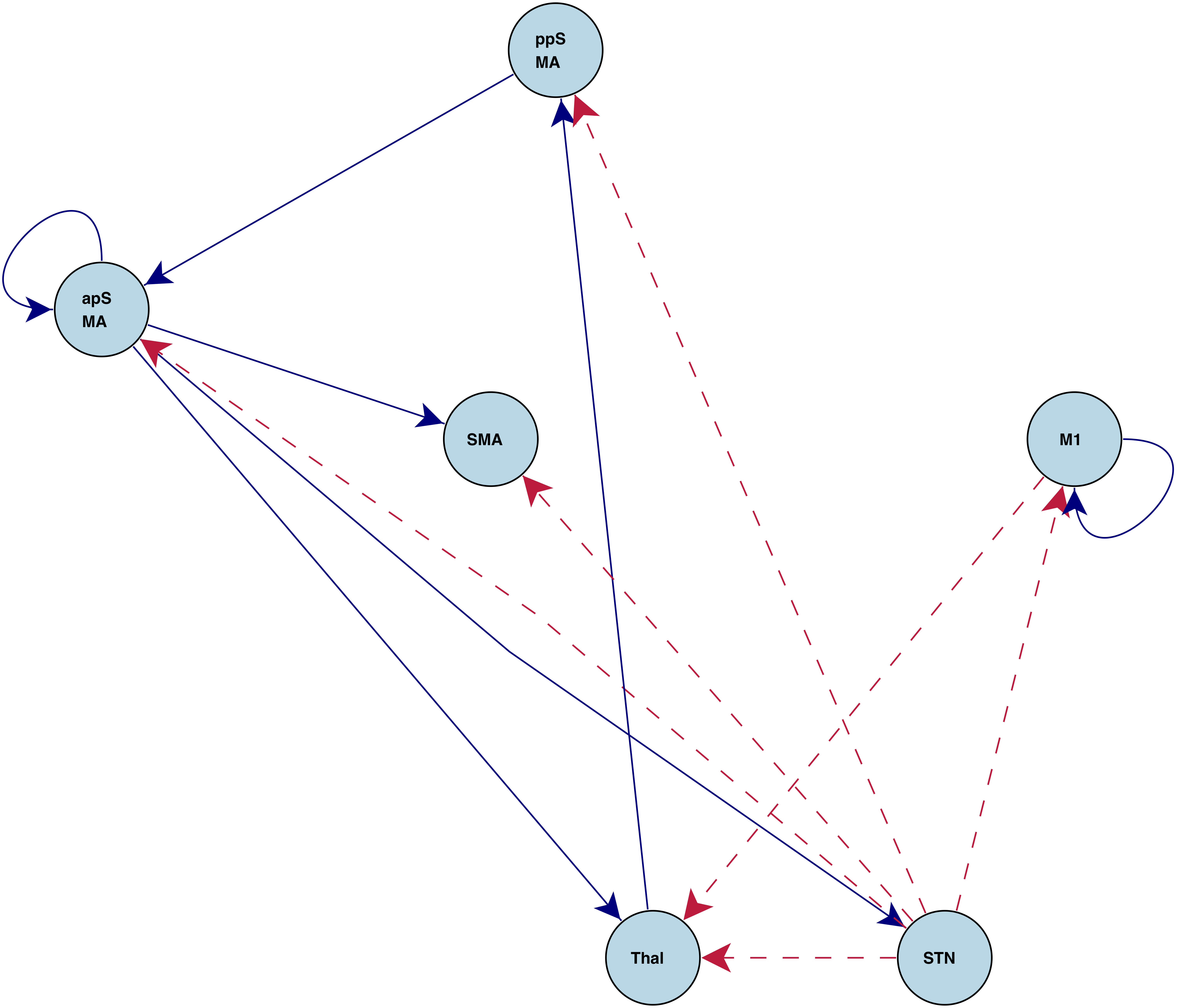"Stop" connections

Better understanding of brain mechanisms

## Resting-state ConnectionsOurs (A) close to DCM (C), different from correaltions (B)

## Real Data: 264 Brain Regions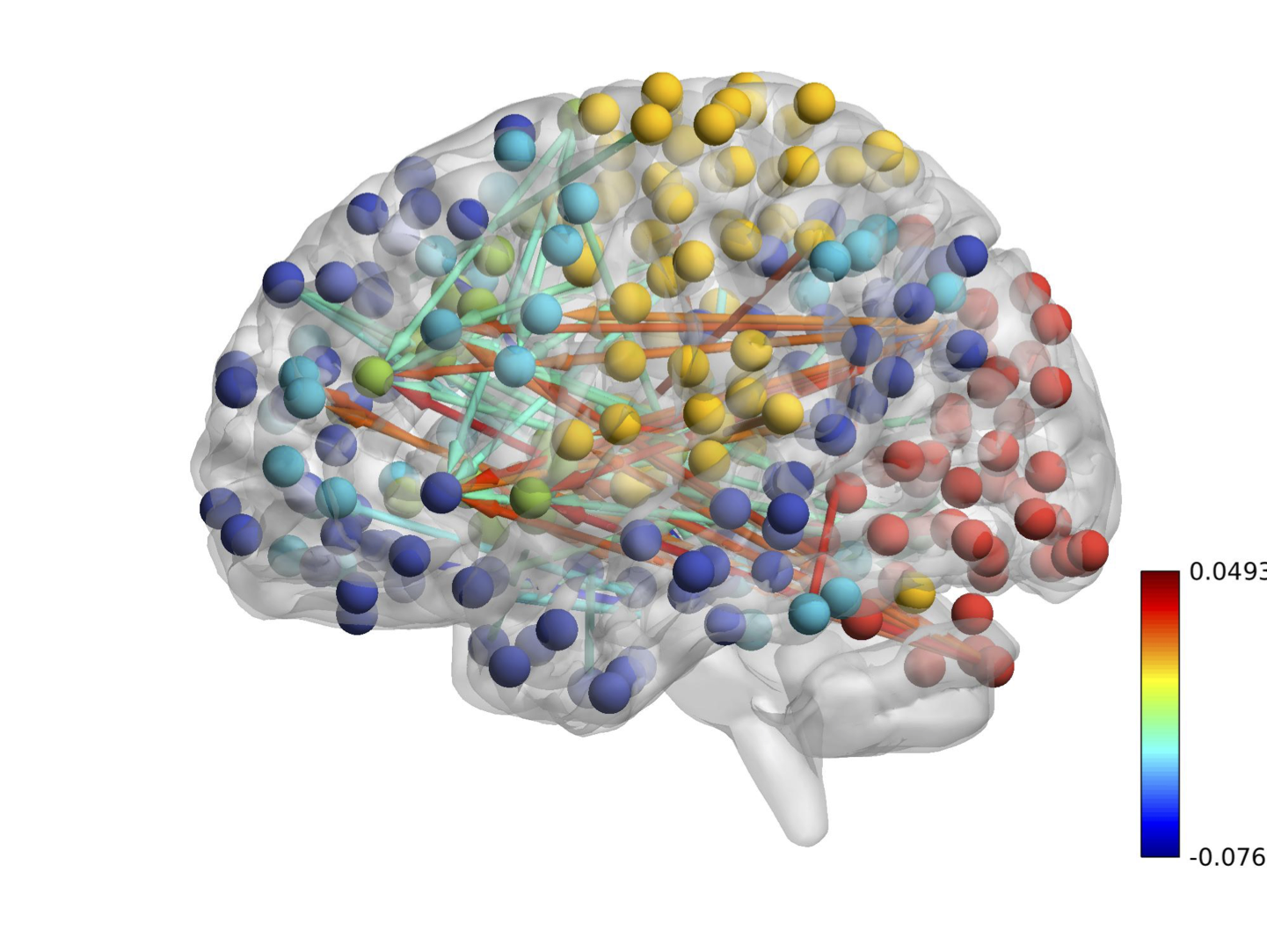CDN uncovers a large-scale brain network

## Discussion

• Joint optimization method for infer ODE networks
• Flexible models for observations from causal ODEs
• Computationally efficient for large-scale modeling
• PyPI pacakge: cdn-fmri